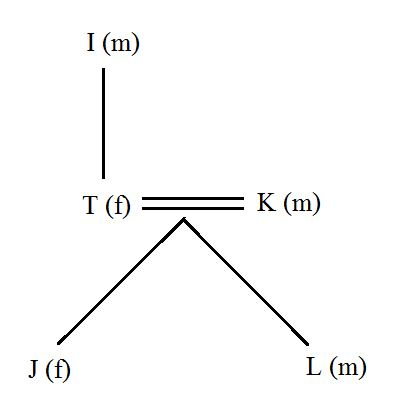# Reasoning Questions and Answers for Bank Exams PDF

0
10243

Reasoning Questions and Answers for Bank Exams PDF:

You can practice Banking Previous year solved papers

Instructions:
If A + B means A is the father of B.
If A × B means A is the sister of B.
If A $B means A is the wife of B. If A % B means A is the mother of B. If A ÷ B means A is the son of B. Question 1: What should come in place of question mark to establish that J is brother of T in the expression? J ÷ P % H ? T % L a) × b) ÷ c)$
d) Either ÷ or ×
e)Either + or ÷

Question 2: Which of the given expressions indicates that M is daughter of D?

a) L % R $D + T × M b) L + R$ D + M × T
c) L % R % D + T ÷ M
d) D + L $R + M × T e)L$ D ÷ R % M ÷ T

Question 3: Which of the following options is true if the expression ‘I + T % J × L ÷ K’ is definitely true?

a) L is daughter of T
b) K is son-in-law of I
c) I is grandmother of L
d) T is father of J
e)J is brother of L

Question 4: Which of the following expressions is true if Y is son of X is definitely false?

a) W % L × T × Y ÷ X
b) W + L × T × Y ÷ X
c) X + L × T × Y ÷ W
d) W $X + L + Y + T e)W % X + T × Y ÷ L Question 5: What should come in place of question mark to establish that T is sister-in-law of Q in the expression. R % T × P ? Q + V a) ÷ b) % c) × d)$
e)Either $or × Solutions: 1) Answer (A) J ÷ P % H ? T % L => T is the mother of L and J is the brother of H Now, for J to be brother of T, H must be either sister or brother of T. => ‘x’ is the correct symbol here. 2) Answer (D) In option B, D + M is given, wich implies that D is the father of M. Now, if M is a girl, then it can be established that M is the daughter of M. M x T => M is a sister of T => M is a girl Hence option B is correct. 3) Answer (B)I + T % J × L ÷ K L ÷ K => L is the son of K J × L ÷ K => J is the daughter of K T % J × L ÷ K => T is the wife of K I + T % J × L ÷ K => I is the father-in-law of K Hence B is the correct answer 4) Answer (D) Given that Y is not a son of X. In option A and B, Y ÷ X is given, which is wrong. Hence A and B are eliminated. In option C, X + L x T x Y is given => X is father of Y, which is wrong. Hence C is eliminated. Similarly E can also be eliminated. Hence, D is the answer 5) Answer (D) T × P ? Q Here, T is the sister of P. So for T to be sister-in-law of Q, P must be Q’s wife. Hence the correct symbol is ‘$’.